Question

# Suppose a 0.270 -kg mass on a spring-loaded gun that has been compressed 0.210 m has...

Suppose a 0.270 -kg mass on a spring-loaded gun that has been compressed 0.210 m has elastic potential energy of 24.0 J. How high above the spring's equilibrium point can the gun fire the mass if the gun is fired straight up?

From the conservation of energy

Initial elastic potential energy = Final gravitational potential energy

there fore 24 J = mgh

height above the equilibrium point h = 24/mg = 24/(.270*9.8) = 9.07 meters

#### Earn Coins

Coins can be redeemed for fabulous gifts.

Similar Homework Help Questions
• ### 9. A spring-loaded toy gun is used to shoot a ball of mass m-2.50 kg straight...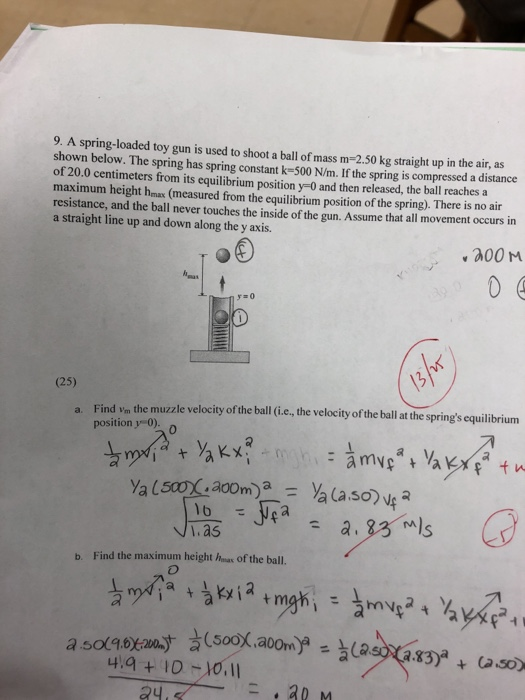9. A spring-loaded toy gun is used to shoot a ball of mass m-2.50 kg straight up in the air, as shown below. The spring has spring constant k-500 NI/m. If the spring is compressed a distance of 20.0 centimeters from its equilibrium position y-0 and then released, the ball reaches a maximum height hmax (measured from the equilibrium position of the spring). There is no air resistance, and the ball never touches the inside of the gun. Assume that...

• ### A spring-loaded toy gun is used to shoot a ball of mass m=1.50 kg straight up in the air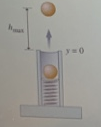A spring-loaded toy gun is used to shoot a ball of mass m=1.50 kg straight up in the air, as shown in (Figur 1) . The spring has spring constant k =667 N/m. If the spring is compressed distance of 25.0 centimeters from its equilbriurn position y = 0 and then released, the ball reaches a maximum height hax (measured from the equilibrium position of the spring). There is no air resistance, and the ball never touches the inside of...

• ### A spring-loaded toy gun is used to shoot a ball of mass m=1.50kg straight up in...

A spring-loaded toy gun is used to shoot a ball of mass m=1.50kg straight up in the air, as shown in (Figure 1) . The spring has spring constant k=667N/m. If the spring is compressed a distance of 23.0 cm from its equilibrium position y=0 and then released, the ball reaches a maximum height hmax (measured from the equilibrium position of the spring). There is no air resistance, and the ball never touches the inside of the gun. Assume that...

• ### A hockey puck of mass m = 0.170 kg is loaded into a spring gun with...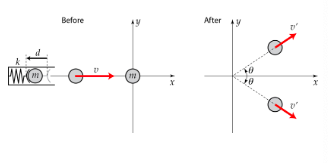A hockey puck of mass m = 0.170 kg is loaded into a spring gun with spring constant k = 306 N/m. The spring is compressed by a distance d = 0.100 m and then released, launching the puck onto a horizontal and frictionless surface of ice with speed v in the positive x-direction. This puck then collides with another puck of the same mass which is at rest at the origin. After the collision the two pucks move away...

• ### 1.A spring-loaded dart gun with a spring constant K= 2000 N/m is used to shoot a...

1.A spring-loaded dart gun with a spring constant K= 2000 N/m is used to shoot a dart with a mass .20kg straight up into the air, and the dart reaches a maximum height of 10 meters. How far did the spring need to be compressed? 2. If i wanted to measure the amount of potential energy in the spring inside my nerf gun, with some nerf darts, what is an easy way i could do it? Explain the steps you...

• ### Fun with a spring gun

A spring-loaded toy gun is used to shoot a ball of mass m= 1.50kg straight up in the air, as shown in the figure. The spring has spring constant k=667 N/m. If thespring is compressed a distance of 25.0 centimeters from its equilibrium position y=0 and then released, the ball reaches a maximum height hmax(measuredfrom the equilibrium position of the spring). There is no air resistance, and the ball never touches the inside of the gun. Assume that all movement...

• ### A dart gun consists of a spring (k = 367 N/m) and a 25.0 g dart....

A dart gun consists of a spring (k = 367 N/m) and a 25.0 g dart. When the dart is loaded into the gun, the spring is compressed 7.0 cm. The gun is aimed straight up and fired. a) How high does the dart go, ignoring air resistance of course? b) How fast is the dart going when it comes back down to the horizontal level at which it was fired?

• ### A spring-loaded launcher is used to shoot a ball of mass 1.50 kg straight up in...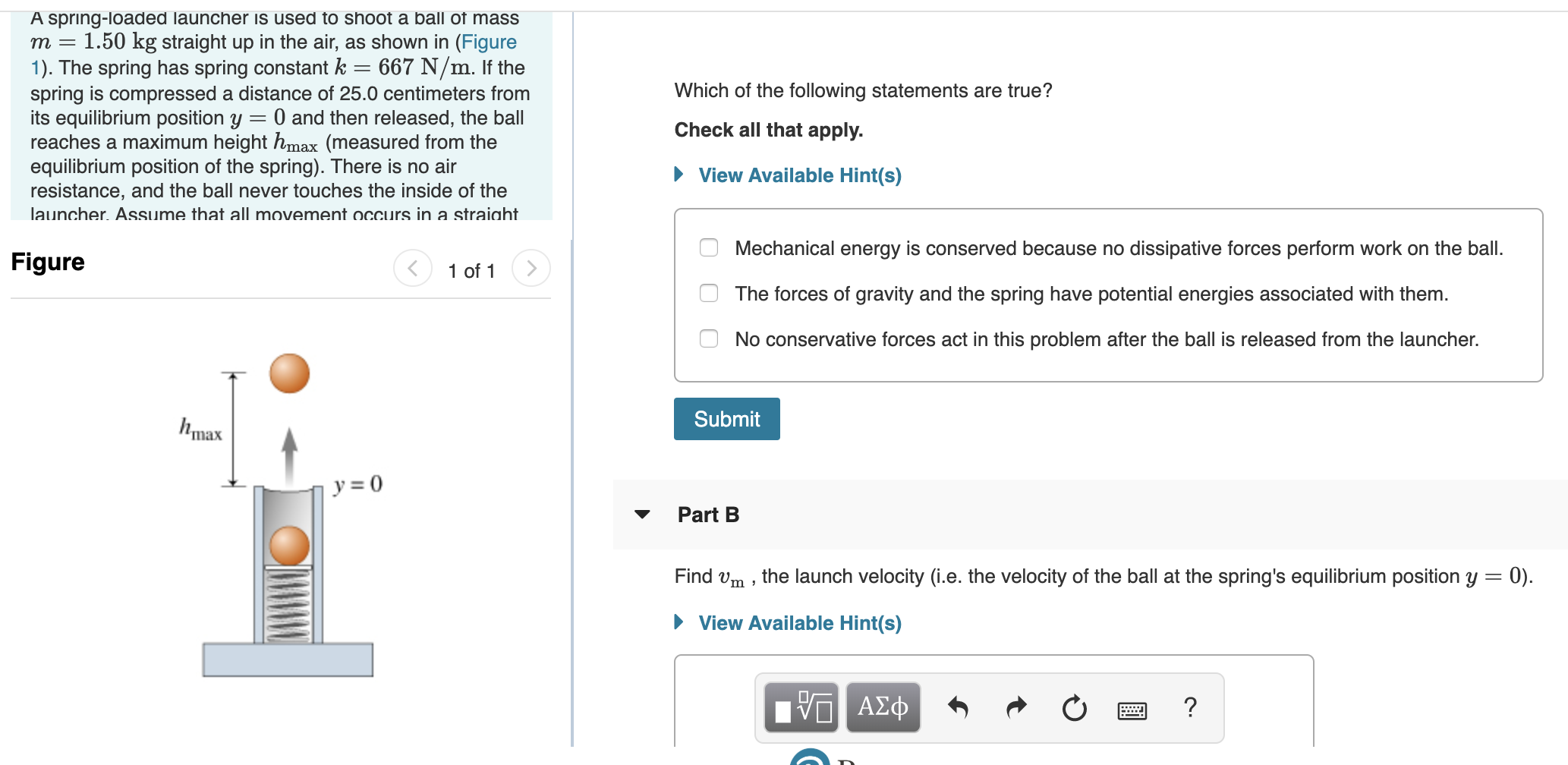A spring-loaded launcher is used to shoot a ball of mass 1.50 kg straight up in the air, as shown in (Figure 1). The spring has spring constant k 667 N/m. If the spring is compressed a distance of 25.0 centimeters from its equilibrium position y = 0 and then released, the ball reaches a maximum height hmax (measured from the equilibrium position of the spring). There is no air resistance, and the ball never touches the inside of the...

• ### 1. 2. A spring-loaded toy gun is used to shoot a ball of mass M straight...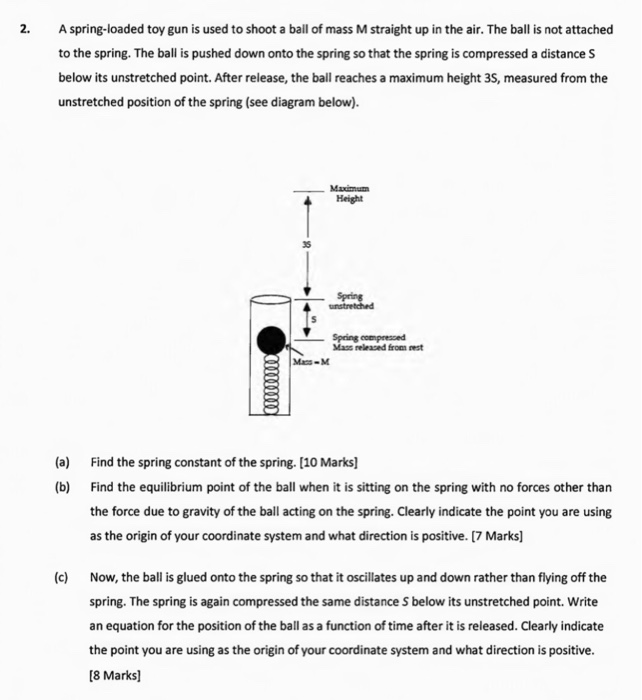1. 2. A spring-loaded toy gun is used to shoot a ball of mass M straight up in the air. The ball is not attached to the spring. The ball is pushed down onto the spring so that the spring is compressed a distance S below its unstretched point. After release, the ball reaches a maximum height 35, measured from the unstretched position of the spring (see diagram below). Maximum Height Spring unstretched Spring compressed Mass released from west will...

• ### solve and show work A block of mass m = 1.91 kg attached to a horizontal...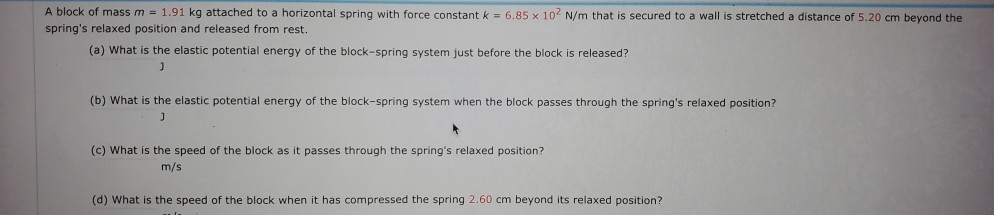solve and show work A block of mass m = 1.91 kg attached to a horizontal spring with force constant k = 6.85 x 10' N/m that is secured to a wall is stretched a distance of 5.20 cm beyond the spring's relaxed position and released from rest. (a) What is the elastic potential energy of the block-spring system just before the block is released? 3 (b) What is the elastic potential energy of the block-spring system when the block...